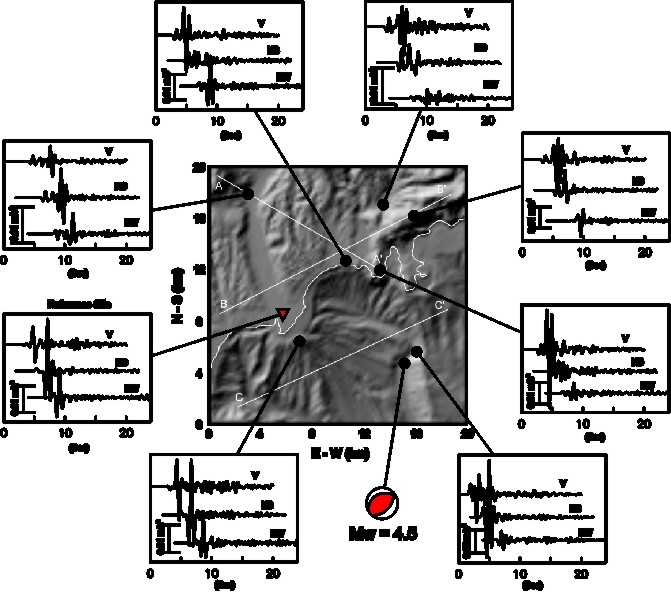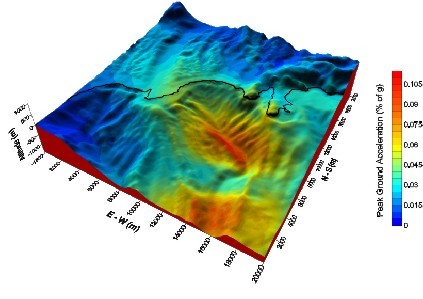;

## Current Work

CONFERENCES

gggggg .

Three-dimensional earthquake simulation (Mw=4.5) in the Nice Region, France

Wave-propagation simulation by a partly-staggered finite-differences method (Cruz-Atienza, 2006) of an inverse earthquake (Mw=4.5) near the coast of Nice (~10 km toward southeast). The source is 8 km depth from the see bottom with seismic moment M0 = 5.96 X 10^15 N*m and the following focal mechanism: f = 243 deg, d = 41 deg and l = 74 deg. The propagation medium is a half-space composed by two different elastic materials: an average crustal solid and see water. Figure 1 shows the topography and bathymetry of the Nice region which was discretized every 50 m to perform numerical simulations (Cruz-Atienza et al., 2006; Cruz-Atienza et al., 2007) (english or french). Figure 2 present a multi-component 3D animation of the velocity field in the free surface (animation by Nahúm Pérez-Campos, Engineering Faculty, UNAM, Mexico). Free surface velocity-deformations are exaggerated 50,000 times in the two horizontal components while 100,000 times in the vertical component.Figure 1 Topography and bathymetry of the Nice region. Figure 2 Free-surface multi-component velocity-field animation.Figure 3 Earth-solid-surface PGA amplification factors computed with (right) and without (left) the sea water layer for four different source frequency content. The reference site is shown in Figure 4 (red triangle). No amplification: yellow contours.Figure 4 Low-pass filtered (fc=1.5 Hz) strong ground motions computed with the sea water layer in different earth-solid-surface locations.Figure 5 Shakemap: earth-solid-surface peak ground accelerations (PGA) expressed as percentage of g (9.81 m/s2), the gravity acceleration. Maximum obtained values correspond to an instrumental intensity equal to I, which implies no potential damage. Simulation considering sea water column.